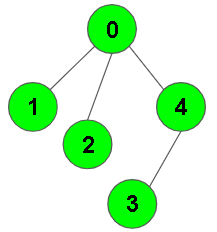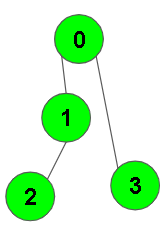XDAYS

:

HOUR

:

MINS

:

SEC

Copied to Clipboard
DFS of Graph
Easy Accuracy: 49.62% Submissions: 100k+ Points: 2

Given a connected undirected graph. Perform a Depth First Traversal of the graph.
Note: Use recursive approach to find the DFS traversal of the graph starting from the 0th vertex from left to right according to the graph..

Example 1:

Input:Output: 0 1 2 4 3
Explanation:
0 is connected to 1, 2, 4.
1 is connected to 0.
2 is connected to 0.
3 is connected to 4.
4 is connected to 0, 3.
so starting from 0, it will go to 1 then 2
then 4, and then from 4 to 3.
Thus dfs will be 0 1 2 4 3.


Example 2:

Input:Output: 0 1 2 3
Explanation:
0 is connected to 1 , 3.
1 is connected to 2.
2 is connected to 1.
3 is connected to 0.
so starting from 0, it will go to 1 then 2
then back to 0 then 0 to 3
thus dfs will be 0 1 2 3.


You dont need to read input or print anything. Your task is to complete the function dfsOfGraph() which takes the integer V denoting the number of vertices and adjacency list as input parameters and returns  a list containing the DFS traversal of the graph starting from the 0th vertex from left to right according to the graph.

Expected Time Complexity: O(V + E)
Expected Auxiliary Space: O(V)

Constraints:
1 ≤ V, E ≤ 104

We are replacing the old Disqus forum with the new Discussions section given below.

### Editorial

We strongly recommend solving this problem on your own before viewing its editorial. Do you still want to view the editorial?

#### My Submissions: